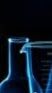1:49 AM
0$(a,b)$ is a couple of twin primes such that $b=a+2$ and $a > 29$. Let $N = 4^b$ and $q$ the quotient which results from the division of $N$ by $a$ and $r$ is the remainder. We calculate $P = (q\bmod b)a+r-1$ Below we prove that $P = 3(10b+1)$ using Fermat's little theorem. $N=16\cdot4^a\equiv64... 2 hours later…3:30 AM 0Let$S = \{ x^2 : x \in \Bbb{Z}\}$. It forms a mult. submonoid of$\Bbb{Z}$. Define$\Omega: \Bbb{Q}^{\times} \to \Bbb{Z}$to be$\Omega(\dfrac{a}{b}) = \Omega(a) - \Omega(b)$. It forms a group homomorphism. So it's kernel$K = \ker \Omega$is the set of all fractions$\dfrac{q_1\cdots q_n}{r...

3 hours later…6:26 AM6:46 AM7:27 AM
Is this question suitable for MSE ?

2 hours later…9:19 AM
[ SmokeDetector | MS ] Link at beginning of body (40): Category Theory: product of mono-arrows is mono?‭ by userx‭ on math.SE

2 hours later…11:10 AM

6 hours later…5:13 PM5:40 PM
I had dupe-hammered this question, but it was inadvertently reopened by another gold-badge holder. I suggest to close the question again, the given answer does not add anything to the solutions given before.@MartinR It is closed. I would recommend deletion.@XanderHenderson Thanks. Agreed.

4 hours later…10:00 PM